# more visualization

Time series

image wrangling

In :
import pandas as pd
import matplotlib.pyplot as plt
% matplotlib inline
from yahoo_finance import Share
import numpy as np

In :
btx=Share('BTX')
df=pd.DataFrame(btx.get_historical('2016-01-01','2016-04-01'))
df['Date']=pd.to_datetime(df['Date'])
df.dtypes

Out:
Adj_Close            object
Close                object
Date         datetime64[ns]
High                 object
Low                  object
Open                 object
Symbol               object
Volume               object
dtype: object
In :
df.index=df['Date']
df=df.drop(['Date','Symbol'],axis=1)

In :
df.head(3)

Out:
Adj_Close Close High Low Open Volume
Date
2016-04-01 2.92 2.92 3.02 2.84 2.90 281100
2016-03-31 2.87 2.87 2.90 2.76 2.83 277800
2016-03-30 2.77 2.77 2.83 2.65 2.70 239900
In :
plt.plot(df.iloc[:,:-1])

Out:
<matplotlib.legend.Legend at 0x7f16d7c191d0>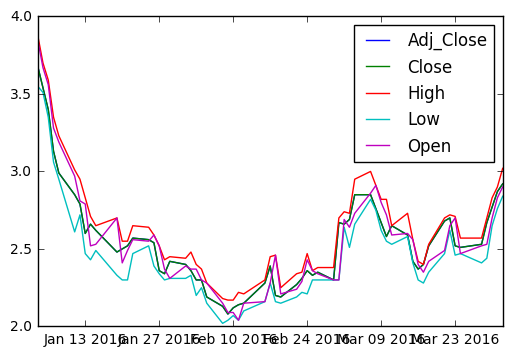## Plotting an inset view¶

In :
plt.plot(df['2016-01']['Open'])
plt.xticks(size=7, rotation=40)

plt.axes([0.55,0.4,0.3,0.45])
plt.plot(df['2016-02']['Open'],color='red')
plt.xticks(size=5, rotation=25)

Out:
(array([ 735997.,  736000.,  736003.,  736006.,  736009.,  736012.,
736015.,  736018.,  736021.]), <a list of 9 Text xticklabel objects>)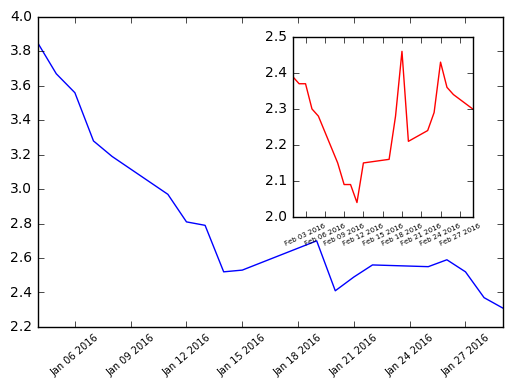## Cumulative Distribution Function from an image histogram¶

• A histogram of a continuous random variable is sometimes called a Probability Distribution Function (or PDF).
• The area under a PDF (a definite integral) is called a Cumulative Distribution Function (or CDF). The CDF quantifies the probability of observing certain pixel intensities.
• The histogram option cumulative=True permits viewing the CDF instead of the PDF.
In :
orig = plt.imread('cat.jpg')
print orig.shape
pixels = orig.flatten()
print len(pixels), pixels.max(), pixels.min()

(194, 259, 3)
150738 255 0


### plt.twinx()¶

• The command plt.twinx() allows two plots to be overlayed sharing the x-axis but with different scales on the y-axis.
In :
# Display a histogram of the pixels
plt.hist(pixels, bins=64, range=(0,256), normed=False,
color='red', alpha=0.3)

# Use plt.twinx() to overlay the CDF
plt.twinx()

# Display a cumulative histogram of the pixels

plt.hist(pixels, bins=64, range=(0,256), normed=True,cumulative=True,
color='blue', alpha=0.3)
plt.title('PDF & CDF (original image)')

plt.show()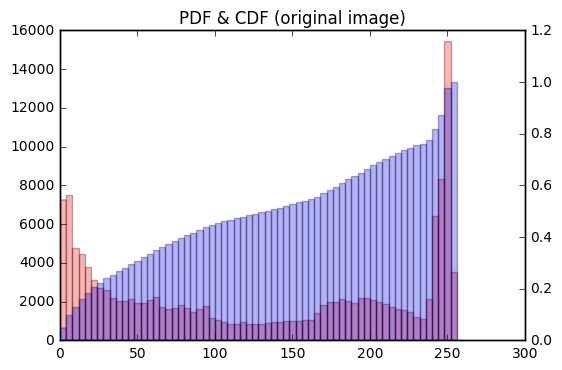## Equlize the image¶

• Histogram equalization is an image processing procedure that reassigns image pixel intensities. The basic idea is to use interpolation to map the original CDF of pixel intensities to a CDF that is almost a straight line. In essence, the pixel intensities are spread out and this has the practical effect of making a sharper, contrast-enhanced image. This is particularly useful in astronomy and medical imaging to help us see more features.

https://en.wikipedia.org/wiki/Histogram_equalization

In :
# Load the image into an array: image

# Flatten the image into 1 dimension: pixels
pixels = image.flatten()

# Generate a cumulative histogram
cdf, bins, patches = plt.hist(pixels, bins=256, range=(0,256), normed=True, cumulative=True)
new_pixels = np.interp(pixels, bins[:-1], cdf*255)

# Reshape new_pixels as a 2-D array: new_image
new_image = new_pixels.reshape(image.shape)

# Display the new image with 'gray' color map
plt.subplot(2,1,1)
plt.title('Equalized image')
plt.axis('off')
plt.imshow(new_image, cmap='gray')

# Generate a histogram of the new pixels
plt.subplot(2,1,2)
pdf = plt.hist(new_pixels, bins=64, range=(0,256), normed=False,
color='red', alpha=0.4)
plt.grid('off')

# Use plt.twinx() to overlay the CDF in the bottom subplot
plt.twinx()
plt.xlim((0,256))
plt.grid('off')

plt.title('PDF & CDF (equalized image)')

# Generate a cumulative histogram of the new pixels
cdf = plt.hist(new_pixels, bins=64, range=(0,256),
cumulative=True, normed=True,
color='blue', alpha=0.4)
plt.show()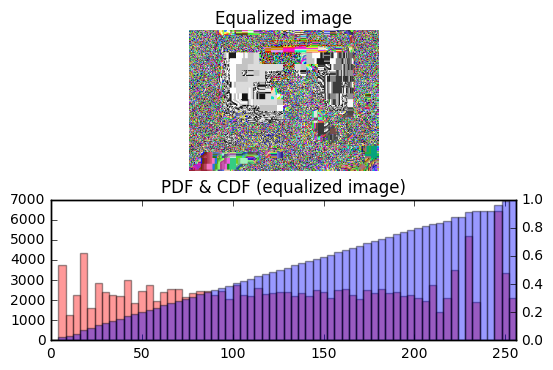## Extracting histograms from a color image¶

• The separate RGB (red-green-blue) channels will be extracted for you as two-dimensional arrays red, green, and blue respectively. You will plot three overlaid color histograms on common axes (one for each channel) in a subplot as well as the original image in a separate subplot.
In :
# Load the image into an array: image

# Display image in top subplot
plt.subplot(2,1,1)
plt.title('Original image')
plt.axis('off')
plt.imshow(image)

# Extract 2-D arrays of the RGB channels: red, blue, green
red, blue, green = image[:,:,0], image[:,:,1], image[:,:,2]

# Flatten the 2-D arrays of the RGB channels into 1-D
red_pixels = red.flatten()
blue_pixels = blue.flatten()
green_pixels = green.flatten()

# Overlay histograms of the pixels of each color in the bottom subplot
plt.subplot(2,1,2)
plt.title('Histograms from color image')
plt.xlim((0,256))
plt.hist(red_pixels, bins=64, normed=True, color='red', alpha=0.2)
plt.hist(blue_pixels, bins=64, normed=True, color='blue', alpha=0.2)
plt.hist(green_pixels, bins=64, normed=True, color='green', alpha=0.2)

# Display the plot
plt.show()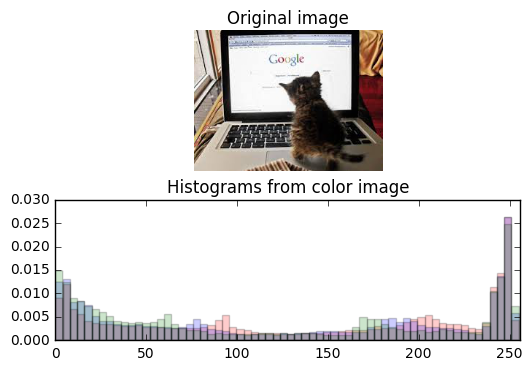## Extracting bivariate histograms from a color image¶

• Rather than overlaying univariate histograms of intensities in distinct channels, it is also possible to view the joint variation of pixel intensity in two different channels.
• The separate RGB (red-green-blue) channels will be extracted for you as one-dimensional arrays red_pixels, green_pixels, & blue_pixels respectively.
In :
# Load the image into an array: image

# Extract RGB channels and flatten into 1-D array
red, blue, green = image[:,:,0], image[:,:,1], image[:,:,2]
red_pixels = red.flatten()
blue_pixels = blue.flatten()
green_pixels = green.flatten()

# Generate a 2-D histogram of the red and green pixels
plt.subplot(2,2,1)
plt.grid('off')
plt.xticks(rotation=60)
plt.xlabel('red')
plt.ylabel('green')
plt.hist2d(red_pixels, green_pixels, bins=(32,32))

# Generate a 2-D histogram of the green and blue pixels
plt.subplot(2,2,2)
plt.grid('off')
plt.xticks(rotation=60)

plt.yticks(size=6)

plt.xlabel('green')
plt.ylabel('blue')
plt.hist2d(green_pixels, blue_pixels, bins=(32, 32))

# Generate a 2-D histogram of the blue and red pixels
plt.subplot(2,2,3)
plt.grid('off')
plt.xticks(rotation=60)

plt.xlabel('blue',size=5,color='r')
plt.ylabel('red')
plt.hist2d(blue_pixels, red_pixels, bins=(32, 32))

plt.subplot(2,2,4)
plt.grid('off')
plt.xticks(rotation=60)
plt.title('orig')
plt.imshow(image)

# Display the plot
plt.show()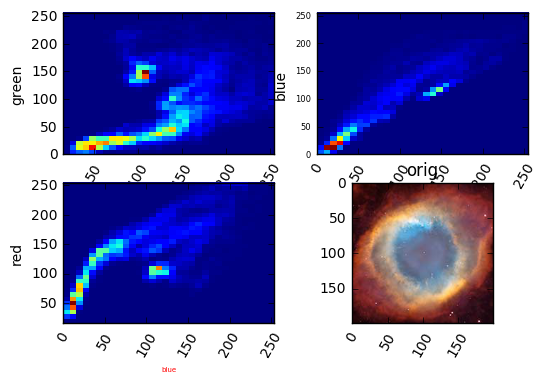In [ ]:


In [ ]:


In [ ]:


In [ ]:


In [ ]: